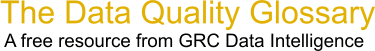Choose Index below for a list of all words and phrases defined in this glossary.

# Regression

index | Index

## Regression - definitions

Regression - The relationship between the mean value of a random variable and the corresponding values of one or more independent variables. A model for predicting one variable from another. A statistical analysis assessing the association between two variables. Regression analysis is a method of analysis that enables you to quantify the relationship between two or more variables (X) and (Y) by fitting a line or plane through all the points such that they are evenly distributed about the line or plane.

[Category=Data Quality ]

Source: iSixSigma, 20 February 2011 11:19:43, http://www.isixsigma.com/index.php?option=com_glossaryThese advertisers support this free service

regression - [statistics] A statistical method for evaluating the relationship between a single dependent variable and one or more independent variables thought to influence the dependent variable. Regression is used to predict the value of the dependent variable or to determine whether an independent variable in fact influences the dependent variable, and to what extent.

[Category=Geospatial ]

Source: esri, 30 July 2012 09:44:15, http://support.esri.com/en/knowledgebase/GISDictionary/term/abbreviationData Quality Glossary.  A free resource from GRC Data Intelligence. For comments, questions or feedback: dqglossary@grcdi.nl